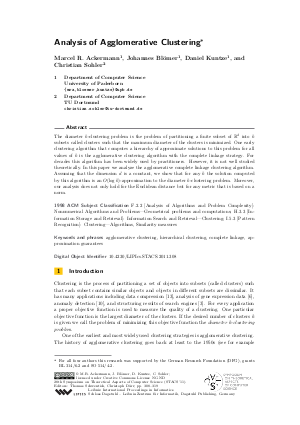Document# Analysis of Agglomerative Clustering

### Authors Marcel R. Ackermann, Johannes Bloemer, Daniel Kuntze, Christian Sohler## File

LIPIcs.STACS.2011.308.pdf
• Filesize: 0.73 MB
• 12 pages

## Cite As

Marcel R. Ackermann, Johannes Bloemer, Daniel Kuntze, and Christian Sohler. Analysis of Agglomerative Clustering. In 28th International Symposium on Theoretical Aspects of Computer Science (STACS 2011). Leibniz International Proceedings in Informatics (LIPIcs), Volume 9, pp. 308-319, Schloss Dagstuhl - Leibniz-Zentrum für Informatik (2011)
https://doi.org/10.4230/LIPIcs.STACS.2011.308

## Abstract

The diameter k-clustering problem is the problem of partitioning a finite subset of R^d into k subsets called clusters such that the maximum diameter of the clusters is minimized. One early clustering algorithm that computes a hierarchy of approximate solutions to this problem for all values of k is the agglomerative clustering algorithm with the complete linkage strategy. For decades this algorithm has been widely used by practitioners. However, it is not well studied theoretically. In this paper we analyze the agglomerative complete linkage clustering algorithm. Assuming that the dimension dis a constant, we show that for any k the solution computed by this algorithm is an O(log k)-approximation to the diameter k-clustering problem. Moreover, our analysis does not only hold for the Euclidean distance but for any metric that is based on a norm.
##### Keywords
• agglomerative clustering
• hierarchical clustering
• approximation guarantees

## Metrics

• Access Statistics
• Total Accesses (updated on a weekly basis)
0